# Vibrational modes of a diatomic linear lattice

A diatomic linear lattice refers to a one-dimensional lattice structure composed of two different types of atoms. In this lattice, the atoms alternate along the linear array.

The vibrational modes of a diatomic linear lattice can be described by considering the motion of the atoms within the lattice. There are two primary types of vibrational modes:

1. Longitudinal modes: In a longitudinal mode, the atoms oscillate back and forth along the direction of the lattice. Both atoms in the unit cell move in the same direction at the same time. This mode is similar to a compression and expansion of the lattice.

2. Transverse modes: In a transverse mode, the atoms oscillate perpendicular to the direction of the lattice. The atoms move in opposite directions, with one atom moving to the right while the other moves to the left. This mode is similar to a shearing or bending of the lattice.

The vibrational modes of a diatomic linear lattice depend on factors such as the masses and bond strengths of the atoms involved. The frequency and energy of these modes can be calculated using mathematical models, such as the harmonic approximation, which assumes simple harmonic motion for the atoms around their equilibrium positions.

The study of vibrational modes in diatomic linear lattices is important for understanding the thermal and mechanical properties of materials, as well as their behavior in different phases, such as solids or liquids. It provides insights into phenomena like thermal conductivity, phonons (quantized lattice vibrations), and the lattice-specific heat capacity.

Let's consider a linear chain of atoms having mass m and M such that M>m. Let 2_n-2, 2_n-1, 2n, 2_n+1 .......are positions of atoms having mass m and M respectively in alternate position. Two atoms are connected by an ideal spring having force constant c. Let U_2n-1, U2n, .....U2n+2 are displacement of atoms 2n-1 .....2n+1 respectively from their equilibrium position. We are considering the elastic response of crystal, the force applied on 2nth atom is linear function of displacement and we are considering the nearesr neighbour interaction.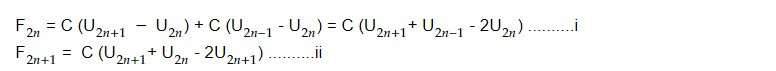From Newton's 2nd law of motion,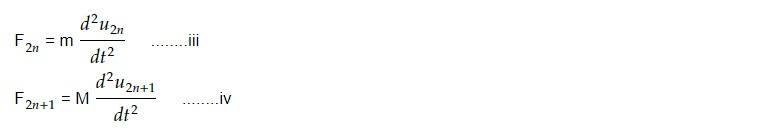On equating i and iii, ii and iv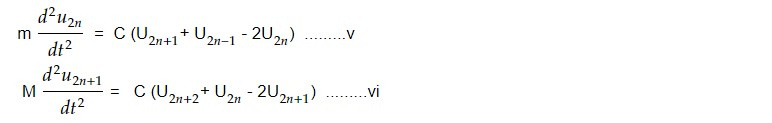we seek the solution of eq v and vi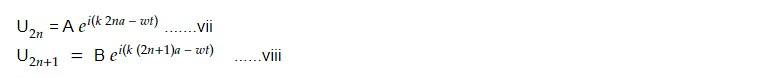Substituting eq vii and viii in vand vi we have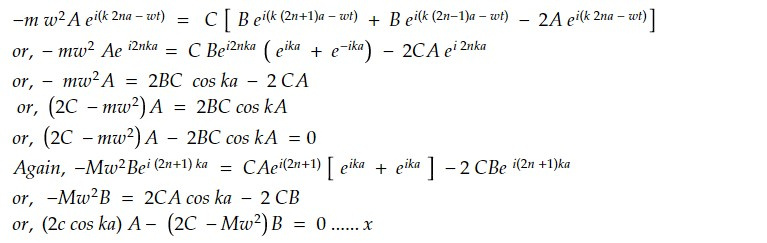Eq ix and x are homogeneous equation with two unknowns so eq ix and x is possible only if determinant of coefficient vanishes.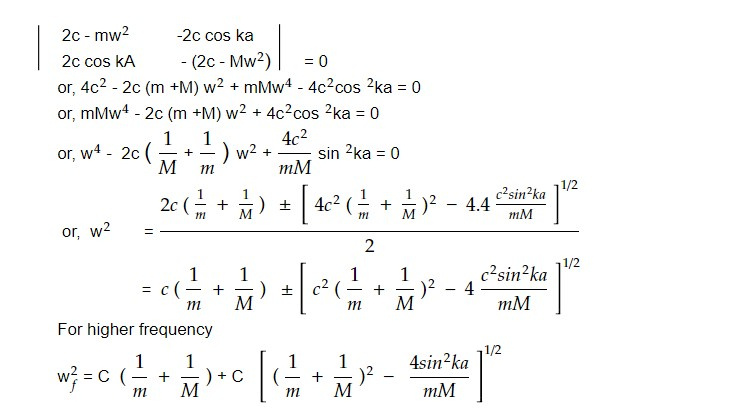At k-> 0, sin ka -> 0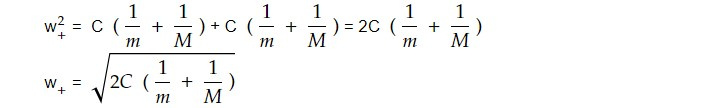At k -> 𝜋/ 2a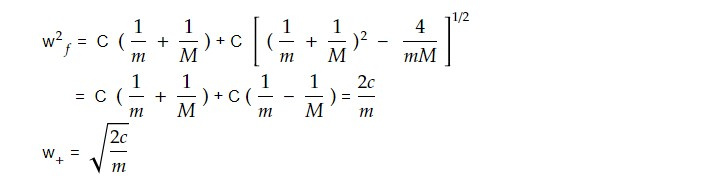For lower frequency,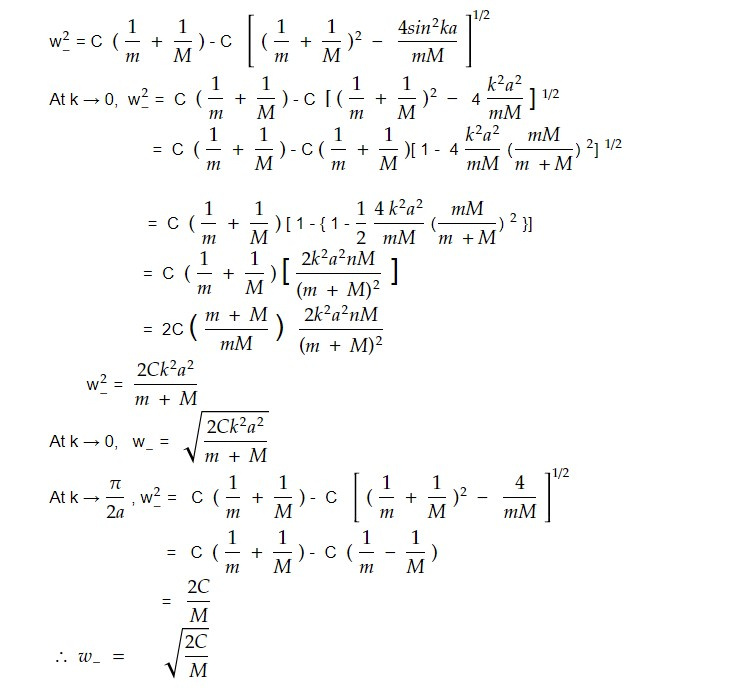Upper branch is called optical branch and lower branch w- is called acoustic branch.

At the region 𝜋/ 2a, the value of w+ is 2C / m and w- is 2C / M. In between frequency 2C / m and 2C / M, there are no wave like solution i.e e^i(kx -wt). The region in which wave like solution is absent in forbidden band gap. The width of band gap depends upon the ratio of masses of two types of atoms i.e band gap depends on ratio M/m. The larger is the ratio, the width the frequency gap. If two masses are equal, the two branches join at k = 𝜋/ 2a and frequency disappear.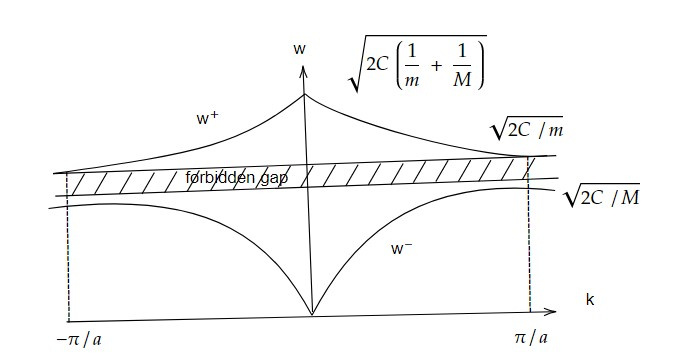This note is a part of the Physics Repository.